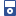Accessibility options:

# Substitution & formulae resources

Show me all resources applicable to

### iPOD Video (8)Substitution and Formulae Part 1
IPOD VIDEO: In mathematics, engineering and science, formulae are used to relate physical quantities to each other. They provide rules so that if we know the values of certain quantities; we can calculate the values of others. In this video we discuss several formulae and illustrate how they are used. This resource is released under a Creative Commons license Attribution-Non-Commercial-No Derivative Works and the copyright is held by Skillbank Solutions Ltd.Substitution and Formulae Part 2
IPOD VIDEO: In mathematics, engineering and science, formulae are used to relate physical quantities to each other. They provide rules so that if we know the values of certain quantities; we can calculate the values of others. In this video we discuss several formulae and illustrate how they are used. This resource is released under a Creative Commons license Attribution-Non-Commercial-No Derivative Works and the copyright is held by Skillbank Solutions Ltd.Substitution and Formulae Part 3
IPOD VIDEO: In mathematics, engineering and science, formulae are used to relate physical quantities to each other. They provide rules so that if we know the values of certain quantities; we can calculate the values of others. In this video we discuss several formulae and illustrate how they are used. This resource is released under a Creative Commons license Attribution-Non-Commercial-No Derivative Works and the copyright is held by Skillbank Solutions Ltd.Substitution and Formulae Part 4
IPOD VIDEO: In mathematics, engineering and science, formulae are used to relate physical quantities to each other. They provide rules so that if we know the values of certain quantities; we can calculate the values of others. In this video we discuss several formulae and illustrate how they are used. This resource is released under a Creative Commons license Attribution-Non-Commercial-No Derivative Works and the copyright is held by Skillbank Solutions Ltd.Substitution and Formulae Part 5
IPOD VIDEO: In mathematics, engineering and science, formulae are used to relate physical quantities to each other. They provide rules so that if we know the values of certain quantities; we can calculate the values of others. In this video we discuss several formulae and illustrate how they are used. This resource is released under a Creative Commons license Attribution-Non-Commercial-No Derivative Works and the copyright is held by Skillbank Solutions Ltd.Substitution and Formulae Part 6
IPOD VIDEO: In mathematics, engineering and science, formulae are used to relate physical quantities to each other. They provide rules so that if we know the values of certain quantities; we can calculate the values of others. In this video we discuss several formulae and illustrate how they are used. This resource is released under a Creative Commons license Attribution-Non-Commercial-No Derivative Works and the copyright is held by Skillbank Solutions Ltd.Substitution and Formulae Part 7
IPOD VIDEO: In mathematics, engineering and science, formulae are used to relate physical quantities to each other. They provide rules so that if we know the values of certain quantities; we can calculate the values of others. In this video we discuss several formulae and illustrate how they are used. This resource is released under a Creative Commons license Attribution-Non-Commercial-No Derivative Works and the copyright is held by Skillbank Solutions Ltd.Substitution and Formulae Part 8
IPOD VIDEO: In mathematics, engineering and science, formulae are used to relate physical quantities to each other. They provide rules so that if we know the values of certain quantities; we can calculate the values of others. In this video we discuss several formulae and illustrate how they are used. This resource is released under a Creative Commons license Attribution-Non-Commercial-No Derivative Works and the copyright is held by Skillbank Solutions Ltd.

Website design by Pink Mayhem, Leicester